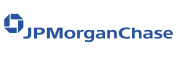New update is available. Click here to update.
Topics

# Sort Elements By Frequency

Easy0/40
Average time to solve is 15m+11 more companies

## Problem statement

You are given a list of a repeated set of integers. Your task for the problem is to return a list of the given elements in decreasing sorted order of their frequency of repetition in the given list with the element with the highest frequency of repetition first and so on.

Note :
``````If two numbers have the same frequency then keep the one that was present before the other in the original given list (array) first.
``````
For Example :
``````Input:  arr[] = {2, 5, 2, 8, 5, 6, 8, 8}
Output: arr[] = {8, 8, 8, 2, 2, 5, 5, 6}

Explanation :
When you sort the array based on the decreasing order of the frequency of repetition of integers in the original array,
you’ll find that the element ‘8’ is the integer with the most repeated values therefore it would be arranged first after which since both 2 and 5 have the same number of repeated
values in the original array but since the 2 arrived first so we will first arrange 2 and then 5 in our resultant array, while would be the last element after sorting here.
``````
Detailed explanation ( Input/output format, Notes, Images )
Constraints :
``````1 <= T <= 10
1 <= N <= 3000
1 <= NUMS[i] <= 10^6

Where 'NUMS[i]' denotes the 'ith' element of the given array.

Time Limit: 1sec
``````
Sample Input 1 :
``````2
7
1 2 3 3 2 1 1
9
1 3 2 2 2 3 4 3 1
``````
Sample Output 1 :
``````1 1 1 2 2 3 3
3 3 3 2 2 2 1 1 4
``````

#### Explanation For Sample Input 1 :

``````When you sort the array based on the decreasing order of the frequency of repetition of integers in the original array,
you’ll find that all different elements ‘1’, ‘2’ and ‘3’ have the same frequency of repetition in the given list of integers but since the order of their arrival in the original array is different
therefore, we arrange them according to that. Hence, the resultant sorted list will become 1 1 2 2 3 3.

When you sort the array based on the decreasing order of the frequency of repetition of integers in the original array,
you’ll find that both elements ‘2’ and ‘3’ have the same frequency of repetition in the given list of integers but since the order of
their arrival in the original array is different therefore we arrange them according to that. While the remaining elements ‘1’ and ‘4’ have different frequencies in the decreasing order of which they can be easily arranged.
Therefore the resultant sorted list will become 3 3 3 2 2 2 1 1 4.
``````
Sample Input 2 :
`````` 1
9
2 5 2 6 9999999 5 8 8 8
``````
Sample Output 2 :
``````8 8 8 2 2 5 5 6 9999999
``````Console